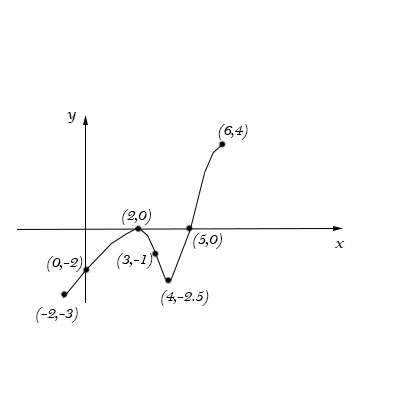# The figure below shows the graph of f ', the derivative of the function f, on the closed interval...

## Question:

The figure below shows the graph of {eq}f ' {/eq}, the derivative of the function {eq}f {/eq}, on the closed interval from {eq}x = -2 {/eq} to {eq}x = 6 {/eq}. The graph of the derivative has horizontal tangent lines at {eq}x = 2 {/eq} and {eq}x = 4 {/eq}. Find the {eq}x {/eq}-value where {eq}f {/eq} attains its absolute minimum value on the closed interval from {eq}x = -2 {/eq} to {eq}x = 6. {/eq} Justify your answer.## Absolute Maximum and Minimum Values of a Function

A function {eq}f(x) {/eq} defined on a closed interval {eq}[a,b] {/eq} attains its absolute maximum or absolute minimum at either one of the endpoints of the interval or at a critical point. A critical point is a point at which the derivative of the function {eq}f'(x) {/eq} is undefined or equal to zero. An absolute maximum is a value {eq}f(c) {/eq} such that {eq}f(c)\geq f(x) {/eq} for all {eq}x {/eq} in the interval {eq}[a,b] {/eq}. Similarly, an absolute minimum is a value {eq}f(c) {/eq} such that {eq}f(c)\leq f(x) {/eq} for all {eq}x {/eq} in the interval {eq}[a,b] {/eq}.

The graph shown is the derivative {eq}f'(x) {/eq}. We know that the original function {eq}f(x) {/eq} is increasing when {eq}f'(x)>0 {/eq} and decreasing when {eq}f'(x)<0 {/eq}. So, the function {eq}f(x) {/eq} is decreasing when the graph shown is below the x-axis and increasing when the graph shown is above the x-axis. So we know that {eq}f(x) {/eq} is decreasing on {eq}(-2,2)\cup(2,5) {/eq} and increasing on {eq}(5, 6) {/eq}

The function is neither increasing nor decreasing at {eq}x=2 {/eq}. Since the function is decreasing everywhere on {eq}(-2,5) {/eq} except for at {eq}x=2 {/eq} where the function is briefly neither increasing nor decreasing, and then the function changes to increasing at {eq}x=5 {/eq}, the function {eq}f(x) {/eq} attains its absolute minimum at {eq}x=5 {/eq}